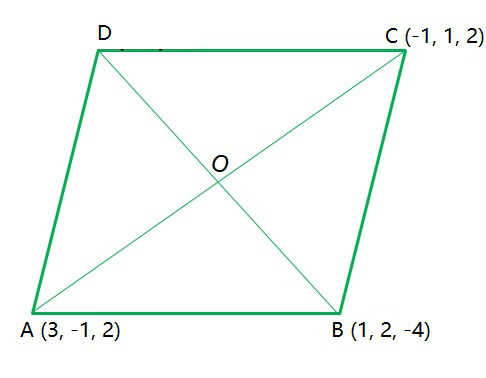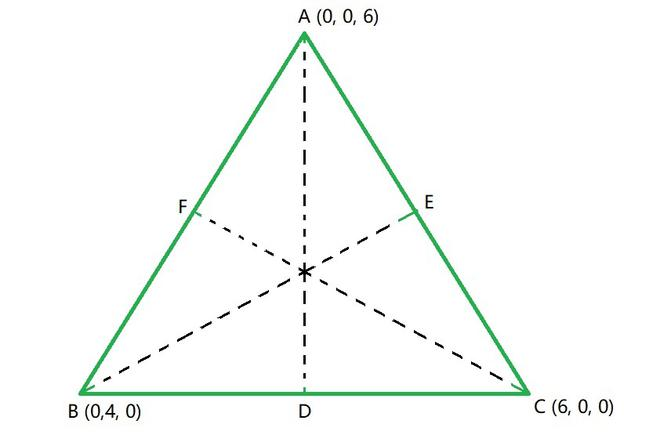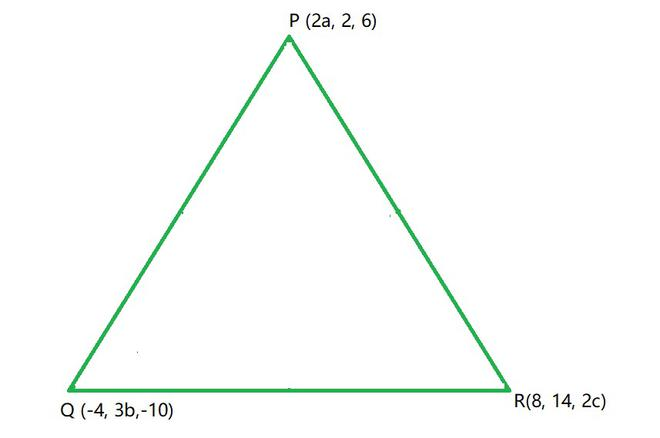Open in App
Not now

# Class 11 NCERT Solutions- Chapter 12 Introduction to three dimensional Geometry – Miscellaneous Exercise on Chapter 12

• Last Updated : 12 Mar, 2021

### Question 1: Three vertices of a parallelogram ABCD are A(3, – 1, 2), B (1, 2, – 4), and C (– 1, 1, 2). Find the coordinates of the fourth vertex.

Solution:

ABCD is a parallelogram, with vertices A (3, -1, 2), B (1, 2, -4), C (-1, 1, 2) and D (x, y, z).Using the property:

The diagonals of a parallelogram bisect each other

Midpoint of AC = Midpoint of BD = Point O

Now, by using Midpoint section formula

Coordinates of O for the line segment joining (x1,y1,z1) and (x2,y2,z2) =So, Coordinates of O for the line segment joining AC == (1, 0, 2) ……………………….(1)

and, Coordinates of O for the line segment joining BD =………….(2)

Using the Eq(1) and Eq(2), we get= 1

x = 1= 0

y = -2= 2

z = 8

Hence, the coordinates of the fourth vertex is D (1, -2, 8).

### Question 2: Find the lengths of the medians of the triangle with vertices A (0, 0, 6), B (0,4, 0), and (6, 0, 0).

Solution:

The vertices of the triangle are A (0, 0, 6), B (0, 4, 0) and C (6, 0, 0).

So, let the medians be AD, BE and CF corresponding to the vertices A, B and C respectively.

D, E and F are the midpoints of the sides BC, AC and AB respectively.Coordinates of mid-point for the line segment joining (x1,y1,z1) and (x2,y2,z2) =So, Coordinates of D for the line segment joining BC =Coordinates of D = (3, 2, 0)

and, Coordinates of E for the line segment joining AC =Coordinates of E = (3, 0, 3)

and, Coordinates of F for the line segment joining AB =Coordinates of F = (0, 2, 3)

By using the distance formula for two points, P(x1,y1,z1) and Q(x2,y2,z2)

PQ =So, AD =AD =and, BE =BE =and, CF =CF == 7

Hence, the lengths of the medians are 7, √34 and 7.

### Question 3: If the origin is the centroid of the triangle PQR with vertices P (2a, 2, 6), Q (– 4, 3b, –10), and R(8, 14, 2c), then find the values of a, b and c.

Solution:

The vertices of the triangle are P (2a, 2, 6), Q (-4, 3b, -10) and R (8, 14, 2c).Coordinates of centroid(0, 0, 0) of the triangle having vertices (x1,y1,z1), (x2,y2,z2) and (x3,y3,z3) =(0, 0, 0) =(0, 0, 0) =So,= 0

a = -2

and,= 0

b =and,= 0

c = 2

Hence, the values of a, b and c are a = -2, b =and c = 2

### Question 4: Find the coordinates of a point on y-axis which are at a distance of 5√2 from the point P (3, –2, 5).

Solution:

Point on y-axis = A (0, y, 0).

Distance between the points A (0, y, 0) and P (3, -2, 5) = 5√2.

Now, by using distance formula,

Distance of PQ =So, the distance between the points A (0, y, 0) and P (3, -2, 5) will be

Distance of AP = √[(3-0)2 + (-2-y)2 + (5-0)2]

= √[32 + (-2-y)2 + 52]

= √[(-2-y)2 + 9 + 25]

5√2 = √[(-2-y)2 + 34]

Squaring on both the sides, we get

(-2 -y)2 + 34 = 25 × 2

(-2 -y)2 = 50 – 34

4 + y2 + (2 × -2 × -y) = 16

y2 + 4y + 4 -16 = 0

y2 + 4y – 12 = 0

y2 + 6y – 2y – 12 = 0

y (y + 6) – 2 (y + 6) = 0

(y + 6) (y – 2) = 0

y = -6, y = 2

Hence, the points (0, 2, 0) and (0, -6, 0) are the required points on the y-axis.

### Question 5: A point R with x-coordinate 4 lies on the line segment joining the points P(2, –3, 4) and Q (8, 0, 10). Find the coordinates of the point R.

[Hint: Suppose R divides PQ in the ratio k : 1. The coordinates of the point R are given by]

Solution:

Let the coordinates of the required point be (4, y, z).

So now, let the point R (4, y, z) divides the line segment joining the points P (2, -3, 4) and Q (8, 0, 10) in the ratio k: 1.

Coordinates of the point which divides PQ in the ratio k : 1 =So, we have= (4, y, z)= 4

8k + 2 = 4 (k + 1)

8k + 2 = 4k + 4

8k – 4k = 4 – 2

4k = 2

k =k =Now, substituting the value we get,

y == -2

z == 6

Hence, the coordinates of the required point are (4, -2, 6).

### Question 6: If A and B be the points (3, 4, 5) and (–1, 3, –7), respectively, find the equation of the set of points P such that PA2 + PB2 = k2, where k is a constant.

Solution:

The points A (3, 4, 5) and B (-1, 3, -7)

Let the point be P (x, y, z).

Now by using distance formula,

Distance of point (x1, y1, z1) and (x2, y2, z2) =So, the distance between the points A (3, 4, 5) and P (x,y,z)) will be

Distance of PA = √[(3-x)2 + (4-y)2 + (5-z)2]

Distance of PB = √[(-1-x)2 + (3-y)2 + (-7-z)2]

As, PA2 + PB2 = k2

[(3 – x)2 + (4 – y)2 + (5 – z)2] + [(-1 – x)2 + (3 – y)2 + (-7 – z)2] = k2

[(9 + x2 – 6x) + (16 + y2 – 8y) + (25 + z2 – 10z)] + [(1 + x2 + 2x) + (9 + y2 – 6y) + (49 + z2 + 14z)] = k2

9 + x2 – 6x + 16 + y2 – 8y + 25 + z2 – 10z + 1 + x2 + 2x + 9 + y2 – 6y + 49 + z2 + 14z = k2

2x2 + 2y2 + 2z2 – 4x – 14y + 4z + 109 = k2

2x2 + 2y2 + 2z2 – 4x – 14y + 4z = k2 – 109

2 (x2 + y2 + z2 – 2x – 7y + 2z) = k2 – 109

(x2 + y2 + z2 – 2x – 7y + 2z) =Hence, the required equation is (x2 + y2 + z2 – 2x – 7y + 2z) =My Personal Notes arrow_drop_up